# At the end, Machine-Learning is all about Optimization : ft.Gradient Descent 👍Fig-1:Caution:So many ups and downs ahead…chill that is part of life 😉

#### Note Before diving into the blog:

Reader should understand Calculus ,especially differentiation up to an order of 2 and must have some knowledge of Linear-Regression algorithm and its evaluation metrics like MSE and RMSE.

### Bird’s-eye view of the Blog:

• Part-1: Over of Loss-Function and Objective behind Optimization.
• Part-2: Theory behind Gradient Descent(Direction and Step-size).
• Part-3: Update Equations.
• Part-4: Challenges with Gradient Descent.
• Part-5: Python implementation of SGD using Boston-House Pricing Data.

#### Short intro of How Optimization works:

We all know Machine-Learning is all about solving complex optimization problems, it can be a simple linear-regression with few weights or a Deep Neural-Network with millions of weights to train.Here training the weights essentially means minimizing the Loss-function(through which we measure our model’s performance)by finding the optimal weights,that represents a mathematical solution to the optimization problem using Gradient Descent.

Now that you have got an idea about optimization, Let us dive deep into it .😎

### a. Overview of Loss-function:

As we humans can visualize only in 3-D and for the sake of simplicity, let us assume we have only two weights to train. In practice we will have millions of weights to train. Let us see how the loss-function looks like with only two parameters to train.

We can see a nice bowl like structure ,with z-axis representing the value of loss-function for particular values of weights which are represented by x and y axes.

### b. Objective behind Optimization :

Our main purpose of optimization is finding the particular values of weights in x-y plane for which the value of loss-function is minimum. Such particular values of weights are called as Optimal weights or minima for the loss-function in literature.

Minima of Loss-function intuitively means,finding the weights at the bottom-most point of the contour of a loss-function,where the Loss-function has its minimum value.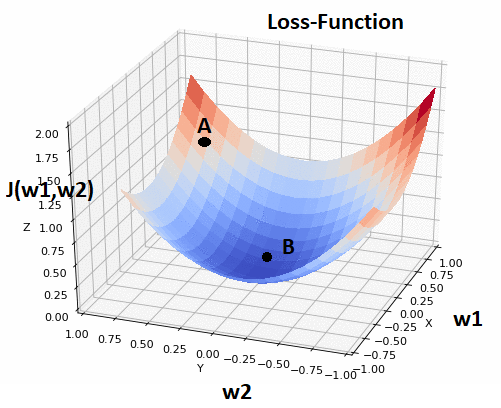Fig-3:Loss function with minima at Point B

We initialize weights randomly at point A,the weights at point A will give very high loss-value which in turn makes our model to perform very badly. From point A, we need to navigate to the point B where the Loss is minimum and find the values of weights at point B,which are our required optimal weights.

Important : Here minima in general refers to the Global minima of the Loss function, there is one more type of minima called Local minima which we will discuss further in this blog.

### How to Navigate to the bottom of the Valley??????Fig-4: Minima is not that easy to reach 😜

### Part-2:

Gradient Descent to the Rescue!!!!!!

Two most important parameters that decide our path to minima are:

1. Direction of in which we should descent to the minima.

2. Size of the step we should take during the descent.

Let us see how do we decide the Direction:

Now that we are at point A in the loss-function contour(Fig-3), we can define a plane that is tangential to that point. In higher dimensional space we will have hyper-plane which is hard to imagine and let us stick to our 3-D visualization we are following till now. Then we will have infinite number of directions to move in x-y plane from that point A. Out of all possible directions, precisely one direction will give us the direction in which the function has the steepest ascent. This direction is given by the Gradient at that point. Exact opposite to that is our direction of steepest descent,moving along which brings about the steepest decline in the value of the loss function. This is how the algorithm got it’s name as Gradient Descent.

For better understanding of this direction and gradient concept ,please refers to the khan-academy tutorial link at the end of this Blog 😇.Brilliant Explanation of this concept.

Now that we got the right direction without the help of google maps 😜 and what NEXT?????

Now we got the direction to move , so now we have to decide about the size of the step we must take. The size of the step is dependent on an important parameter called Learning rate which plays an important role in reaching the minima.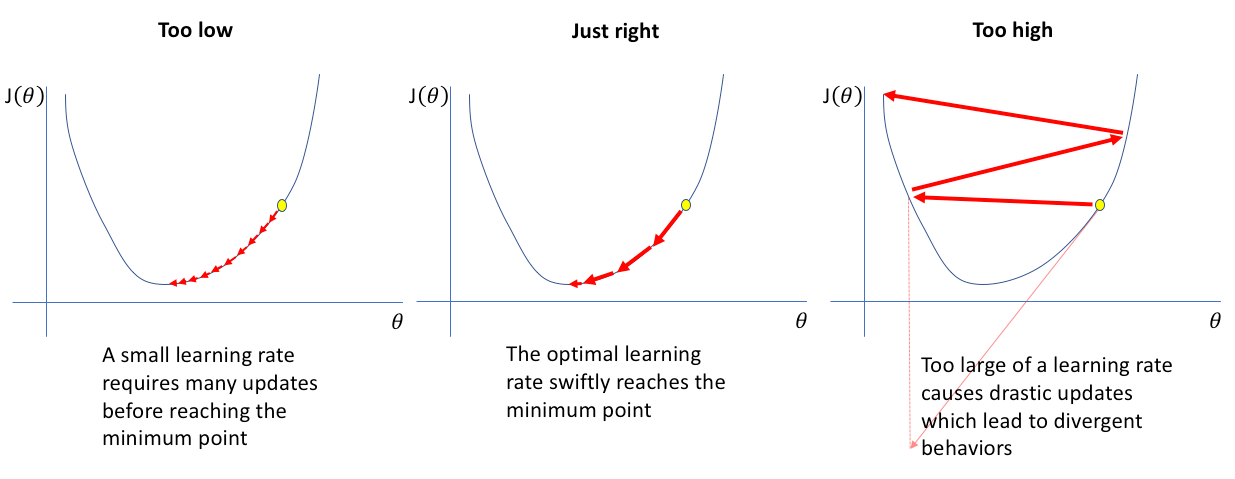Fig-6: Impact of Learning Rate on Descent
• Too low learning rate progresses very slowly and takes lot of time to converge and high risk of getting stuck at local minima (I know you are being impatient with this word)Please wait,you will get clarity in the pre-climax of this blog) 😅
• Initially we can afford to have large learning rate but as we approach minima, the learning rate has to be small. Otherwise we have a problem of overshooting the minima and bounce between the ridges which in turn leads to no convergence.
• Unfortunately there is no specific learning-rate for Optimizers.
• Learning rate can affect the training time by an order of magnitude.
Choosing the best Learning-rate is very crucial in training a model, so How do we choose the best learning-rate then ????
• Some conventional ways of choosing better Learning-Rate
1. Cross-Validation.
2. Hyper-parameter tuning using GridSearchCV.
3. Learning rate with decay after fixed number of iterations can be a very good hack( Decrease the learning rate as we approach minima) which is in technical terms called as Simulated annealing.
Now that we got our direction and step-size, what should we do now?????

Once we got our direction and step-size, we will take a step along the direction opposite to that of direction of gradient at that point and recompute the gradient at whatever position we end up at , we will repeat this process till we reach our required minima.

How do we know whether we reached minima or not????

While the direction opposite to that of direction of gradient which has the steepest descent , the magnitude of the gradient tells us how steep the descent is.At minima the contour is almost flat and we can expect the gradient to be close to zero. Ideally the magnitude of the gradient has to be zero at minima.

Gradient Descent in Action:

In real life, we might can never reach the minima , but we keep dodging in a flat-region in close vicinity of the minima, where the loss is almost minimum.Often we stop our iterations when the change in loss value hasn’t improved much in a pre-defined number like 10 or 15 iterations. When this happens, we can say our training has reached convergence.

A Common Mistake in Understanding the trajectory of Gradient Descent
( i made this mistake when i learnt about Gradient Descent for the first time)

As we see in the above visualization of trajectory of gradient descent, we see that it starts at a point and reaches the minima. However this is a very inaccurate picture of what gradient descent really is. The real trajectory is actually confined to x-y plane which contains our weights which are the free parameters described in x and y directions and doesn’t not involve moving in z-direction at all.

Each point in the x-y plane represents a unique combination of weights, and we need to find the weights at the minima which is at the bottom of the contour.

### How do we update the weights after every iteration?

Update Equation

Here is the basic equation that describes the update rule of Gradient DescentFig-10: Update Equation of weights using GD

This update is performed over every iteration. Here, w is the weight vector that lies in x-y plane. We update these vectors by subtracting the gradient of our loss-function with respect to the weights multiplied by alpha, the Learning-Rate.

Why to subtract the gradient from weights???

As we discussed above, the direction of the gradient gives us the direction in which the loss function has steepest ascent . The direction of descent is exactly opposite to that of direction of gradient, that is why we are subtracting the gradient vector from the weight vector.

This update is simultaneously done for all the weights.

We multiply the gradient vector by learning rate, which is the size of the step we discussed above. One thing we can observe that even if we keep the learning rate constant, the size of the step changes because of the changes in the magnitude of the gradient because of the decrease in the steepness as we approach minima and we tend to take small steps towards minima.

### Challenges with Gradient Descent:

1. Local minima (finally the wait is over 😆)

So far the story of Gradient Descent seems to be happy one but i am going to spoil your happiness.Till now what ever the contour of loss functions we witnessed don’t really exists? So sad right!!!! have a look at the below figure.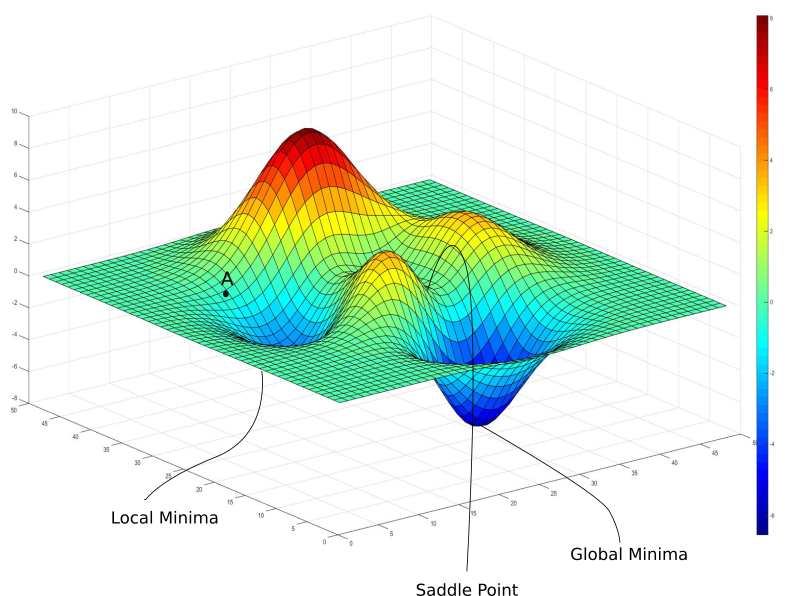Fig-11: Contour of a complex loss function

Not every model we train is a linear regression and Deep neural networks are more complicated functions with lots of non-linear transformations.

The resultant loss function can no more be a nice bowl like structure with only one minima to converge. Loss functions for deep neural networks are hardly convex(always curving upwards)

In the above figure, we have a local minima where the gradient is also zero but we knew that is not the lowest value of loss-function we can achieve,which is the point at global minima. Let us say we initialize the weights at point A(Fig-8),then we will converge to local minima and there is no way we can get out from that local-minima.

Because Gradient descent is driven by the gradient, which will be zero at any minima.

• Minimum in a local region is called Local-minima.
• When we take Minimum globally, that is across the whole region is called Global Minima.
So, Reaching Global minima is our primary Task

#### 2. Saddle Points

It is not only the local minima at which the gradient is zero , there is one more dangerous region called Saddle point.(See the below figure)

A saddle point is just like the saddle of the horse

#### How do we interpret the saddle point????

If we can see,saddle point at the intersection is minima in one direction(x) and local-maxima in another direction. Because of this Gradient Descent keep dodging in this region which will give us the wrong impression that we converged to minima.

### How to tackle these Challenges???

• Randomness will help us in escaping local minima and saddle points.
Randomness ????

Till now in gradient descent, the loss-function is created by summing loss over all the data-points in the training data. In this way we will get stuck in local minima and saddle points. A way to help Gradient Descent to escape these dangers is Stochastic Gradient Descent(SGD).

In SGD, instead of taking all the data-points in the training data we will take only one randomly sampled data point without replacement and compute the loss function and update our weights.

Since each data-point is stochastically chosen( induces randomness), this method is called SGD but in our earlier approach we used all the data points as a single batch, So that method is called Batch Gradient Descent.

The update rule is modified accordingly.Fig-13: Update Equation of weights for SGD
How does randomness will help???

Since we are choosing a point randomly, that point may not lie around a region of local-minima in the contour of the one-example-loss,which allows us move away from it.

Even if we get stuck in a minima for the “one-example-loss”, the loss landscape for the “one-example-loss” for the next randomly sampled data point might be different, allowing us to keep moving.

So, does this mean in practice, should we always perform this one-example stochastic gradient descent????

The answer is a big NO. Though from a theoretical standpoint, SGD performs well but it is not computationally viable as it is required to compute the loss function sequentially step by step.

So How do we tackle this issue of computational viability???

Here we are in between to extremes, So we need to do a balancing act. Instead of using single data-point or entire data-set, we will pick a fixed number of data-points randomly and compute the loss function and update our weights. This method of picking a fixed number of data-points randomly is called mini-batch SGD.

So finally we got three variants of Gradient Descent

• Batch Gradient Descent.
• Stochastic Gradient Descent.
• Mini-Batch Gradient Descent.

### Implementation of Stochastic Gradient Descent for Linear Regression in Python

We are using Classic Boston Housing price prediction Data for this implementation.

More details about this Data set can be found here:

https://www.kaggle.com/c/boston-housing

In precise, given some predictive variables we need to train a Regression-model that predicts the price of the house in Boston region with least RMSE(root mean squared error).

• First we are scaling our data using min-max scale which helps gradient descent to converge faster and we split our data into train and test randomly.
#standardizing the datafrom sklearn.preprocessing import MinMaxScaler
#standardizing the columnsdata = MinMaxScaler().fit_transform(boston_data)
#splitting the data into train and test setsfrom sklearn.model_selection import train_test_splitx_train,x_test=train_test_split(data,test_size=0.3,random_state=123)
• Next we are computing the optimal weights by updating the weights after every iteration using stochastic gradient descent with learning rate equals to 0.01 and number of iterations as 100.
#utility function for predictionsdef pred_price(row, weights):    y_pred = weights    for i in range(len(row)-1):        y_pred += weights[i + 1] * row[i]    return y_pred
#computing optimal weights using gradient descentdef weights_sgd(train, learning_rate, n_epoch):    w = [0.0 for i in range(len(train))]     #number of weights will be equal to number of features    for epoch in range(n_epoch):        for row in train:            y_pred = pred_price(row,w)            error = y_pred - row[-1]            w = w-learning_rate * error             #updating the weights after every iteration            for i in range(len(row)-1):               w[i + 1] = w[i + 1] - learning_rate * error*row[i]              return w#computing the optimal weights   optimal_w = weights_sgd(x_train,0.01,100)T
Here are the optimal weights we got:[0.23074803498670263, -0.057472150434689236, 0.07594095408862375, -0.0417915764508294, 0.034273112789058396, -0.012318945415060211, 0.43070701300257047, 0.03260465927841714, -0.04183764966217743, 0.03747744653325749, -0.06710886450470752, -0.15180704686436672, 0.1416836597740854, -0.27684423645993567]
• Next we use the computed optimal weights to predict the price of the house on test data.(Here weights are the coefficients of equation of hyper-plane that best fits the training data)
#implementing sgd on linear-regressiondef linear_regression_sgd(train, test, learning_rate, n_epoch):    predictions = list()    coef = weights_sgd(train, learning_rate, n_epoch)    for row in test:        y_pred = pred_price(row, coef)        predictions.append(y_pred)    return(predictions) #predicting the values in test-data   y_pred_sgd = linear_regression_sgd(x_train,x_test,0.01,100)
• Computing MSE and RMSE to check the performance of our model.
#getting the actual values of the pricesy_test =[]for i in range(len(x_test)):    price = x_test[i][-1]    y_test.append(price)    #as we did min-max scaling initially,we converting back to original pricesy_test_final = [((i * 45)+5) for i in y_test]y_pred_final = [((i * 45)+5) for i in y_pred_sgd]
from sklearn.metrics import mean_squared_error
#computing the MSEmse_sgd = mean_squared_error(y_test_final,y_pred_final)
#computing RMSErmse_sgd = mse_sgd**0.5
#printing the final resultsprint("Mean squared error : {}".format(mse_sgd))print("Root Mean Squared Error: {}".format(mse_sgd**0.5))
• Let us see the plot between the actual price and predicted price for test data for better understanding.
#ploting plt.figure(figsize=(10,8))sns.set_style('whitegrid')sns.regplot(x=pd.Series(y_test_final),y=pd.Series(y_pred_final))plt.xlabel("Prices: $Y_i$")plt.ylabel("Predicted prices: $\hat{Y}_i$")plt.title("Prices vs Predicted prices: $Y_i$ vs $\hat{Y}_i$")plt.grid(True)plt.show()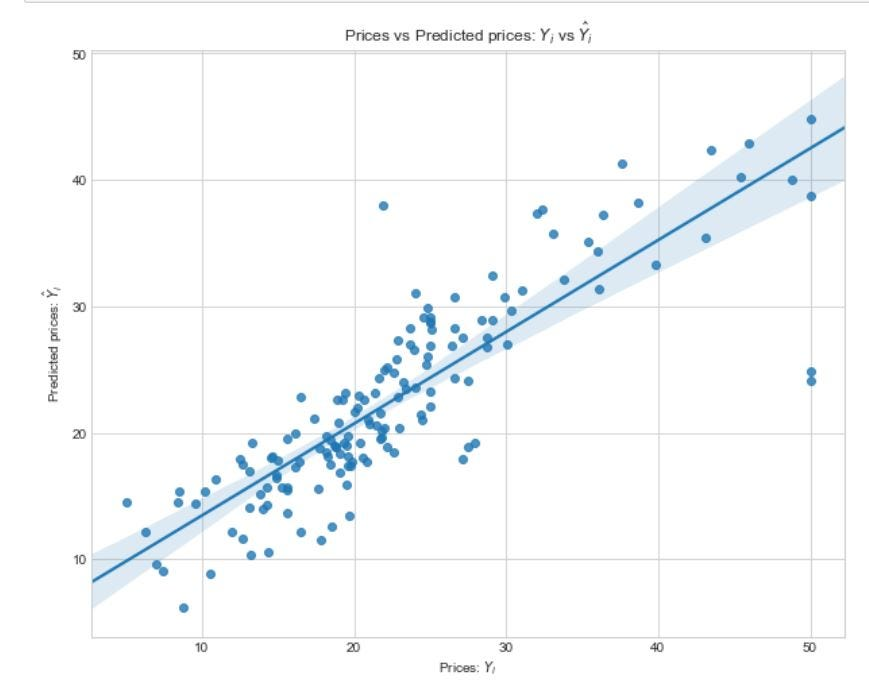Fig-15:Plot b/w actual and predicted prices
• Let us check the distribution of errors that we got with SGD implementation (Here error is the difference b/w the actual price and predicted price)
#checking the distribution of errorsy_error_SGD  = pd.Series(y_test_final)-pd.Series(y_pred_final)
#plotingsns.set_style('whitegrid')plt.figure(figsize=(10,8))sns.kdeplot(np.array(y_error_SGD), bw=0.5)plt.show()
• The distribution of errors is close to Normal Distribution which is sign of good Regression model.

### Further Improvements:

• Gradient descent is a First Order Optimization Method.As it only takes first order derivatives of the loss function, it won’t take curvature of the loss-function into consideration.
• Gradient Descent can tell whether the loss is declining and how fast, but cannot differentiate between whether the curve is a plane, curving upwards or curving downwards.
Pathological Curvature can be a problem in reaching minima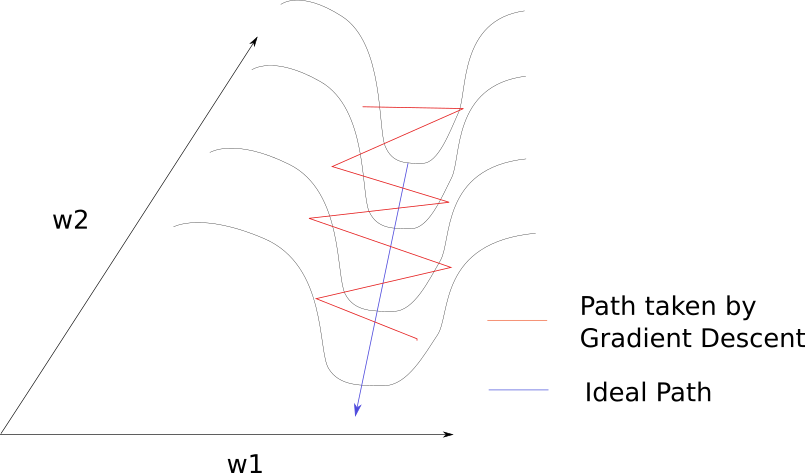Fig-17: Effect of Curvature in reaching minima

See how, Gradient Descent is bouncing along the ridges and taking a lot of time converge to the minima,because of the more steepness in the direction w1, the gradient will move more in the direction of w1 but minima lies along the direction of w2.

• We might think using low-learning rate might solves this problem of bouncing between the ridges but it spells a trouble because of the steepness we already facing the problem slow convergence and using low-learning rate makes it even slower there by giving the wrong impression of no convergence or a local-minima.
• To tackle this issue of pathological curvature ,we need the help of second-order derivatives which will take curvature into consideration.(To know further about this,please refer to the Khan-academy tutorial at the end of this blog)

### Conclusions:

• In optimization using Gradient Descent , the direction and Learning-rate plays an important role in reaching minima.
• Choosing the optimal learning rate is important in both reaching minima as well controlling the training time.
• Major challenges in attaining global minima comes from local-minima and saddle-point.
• Randomness helps us in tackling the challenges of Local-minima and saddle point.
• When the data set is small and low-dimensional, we can go for Batch-Gradient Descent and when it is large and high dimensional , we can go for mini-batch SGD or SGD.

Hope you enjoyed reading this blog and Thanks for reading….!! Stay tune😃